Next: 1.5.5 Self-organizing maps Up: 1.5 Learning of sensorimotor Previous: 1.5.3 Feed-forward networks as

## 1.5.4 Recurrent neural networks as internal models

This section presents two sets of examples showing how recurrent neural networks can be applied. The first set exploits the recurrent connection to predict a series of sensory states, and the second set uses the relaxation to stable points for an associative task.

Tani (1996) used a partially recurrent neural network with a context layer (figure 1.5) as a forward model for a mobile robot. The robot's environment was separated into paths and intersections by obstacles. Here, the sensory state was a set of distances to obstacles, and the motor command, which was binary, represented the path of choice at an intersection. Using backpropagation-through-time, the recurrent network was trained on series of sensory states and corresponding motor commands. After training, given a sequence of motor commands, the network could predict the resulting sensory state.The trained network was also applied to a planning task. Here, the sequence of motor commands is not known, but the final desired sensory state is known. Tani (1996) solved this problem by defining a cost function based on the difference between the desired state and the predicted state that results from a motor sequence. The motor commands were obtained by minimizing this cost function using gradient descent.

In a simulation of a mobile robot, Jirenhed et al. (2001) also used a recurrent neural network for prediction. Here, the environment contained corridors and corners, but no intersections. The robot had two wheels, whose velocities were the motor commands. Instead of having these motor commands as a network input, they were predicted. The goal of this study was to show that the robot can simulate its movement through the environment. Jirenhed et al. (2001) interpreted this simulation as an emerging `inner world'.

Cruse and Steinkühler (1993) showed that the relaxation in a recurrent neural network can be used to solve the inverse kinematics of a redundant robot arm (which can adopt many postures for a given end-effector position). A simulated robot arm was composed of three line segments in the plane. The geometric relations of the arm-joint positions were put into a redundant set of linear equations,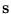=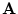, with the unknown state. This set of equations can be represented by a recurrent neural network, interpreting the matrixas a set of weights (figure 1.6). Such a network can complete a partially given state. Any component of the state vectorcan be set equal to the corresponding component of an input vector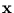, which is fixed in its values. The output is computed by iterating the state,

 si(t + 1) =Aij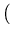[1 - gj]sj(t) + gjxj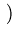, (1.1)

with gj = 1 for input components and gj = 0 for output components. The state relaxes to a stable point, which yields the output values.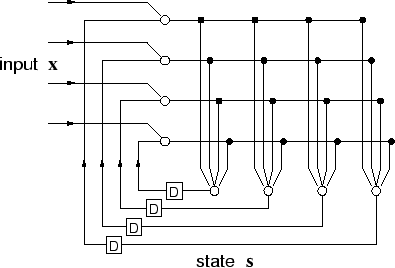This approach was further extended to non-linear equations (using the non-linear functions as activation functions) and to an arm in three-
dimensions with six degrees of freedom (Steinkühler and Cruse, 1998). The application is not limited to a robot arm; a recurrent network can be also built for landmark navigation if the coordinates of the landmarks and of the goal are given (Cruse, 2003b). Cruse (2001) argued that recurrent networks are much more plausible to describe brain function because they allow the animal to obtain an internal state and memory, and thus let the animal escape from being a purely reactive system. In addition, Cruse (2003a) related the recall in recurrent networks, as described above, to the emergence of an internal world.Next: 1.5.5 Self-organizing maps Up: 1.5 Learning of sensorimotor Previous: 1.5.3 Feed-forward networks as
Heiko Hoffmann
2005-03-22